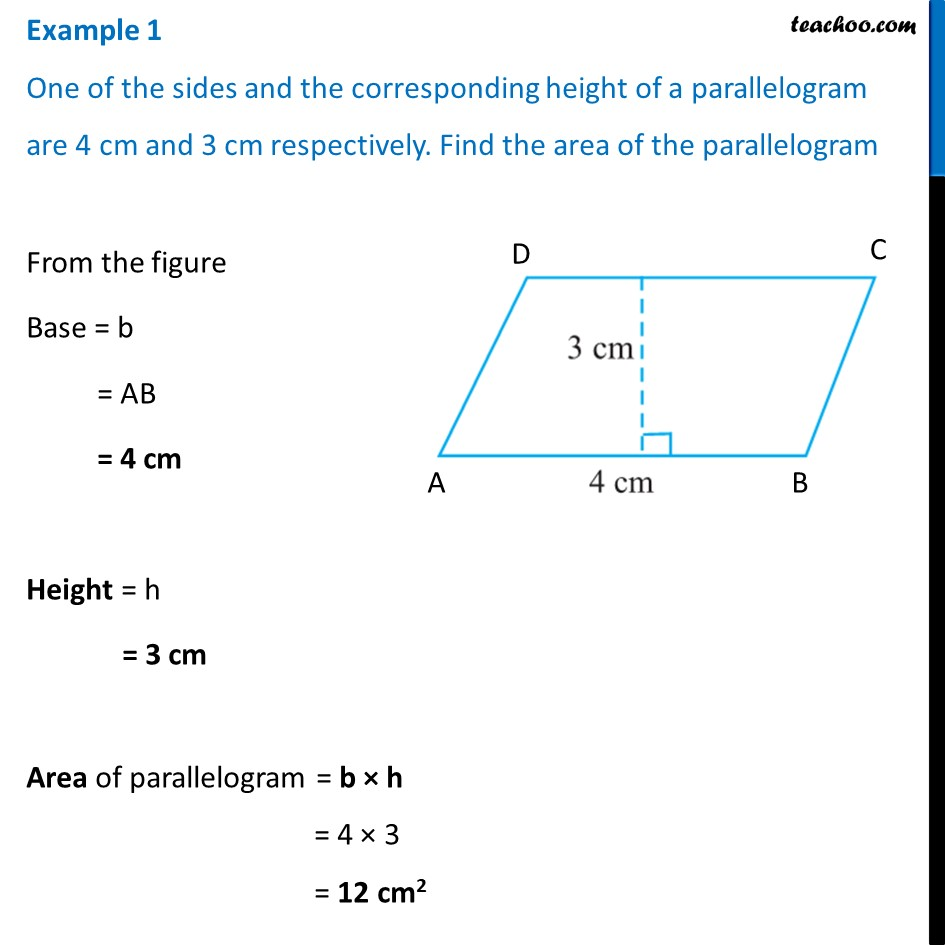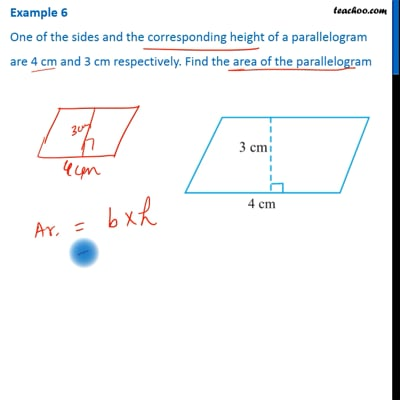Examples

Chapter 9 Class 7 Perimeter and Area
Serial order wiseThis video is only available for Teachoo black users

Learn in your speed, with individual attention - Teachoo Maths 1-on-1 Class

### Transcript

Example 1 One of the sides and the corresponding height of a parallelogram are 4 cm and 3 cm respectively. Find the area of the parallelogramFrom the figure Base = b = AB = 4 cm Height = h = 3 cm Area of parallelogram = b × h = 4 × 3 = 12 cm2